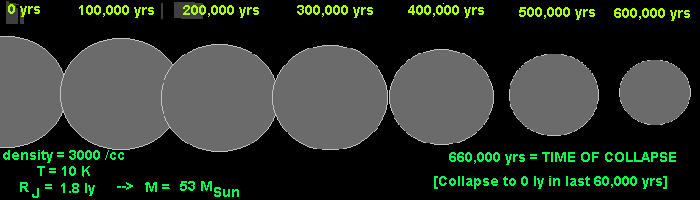## Collapse of Interstellar Gass Clouds

• ### Kinetic Theory of Gases - low density

```Temperature is proportional to the
kinetic theory of molecules of the gas.
KE = 3/2 kT = 1/2 mv2
k = Boltzman Constant
v = mean square speed of molecules
T = absolute temperature```
• ### Gravity and Equilibrium - Virial Theorem

```Kinetic Energy + Potential Energy = Total Energy
Temperature is a measure of internal kinetic energy
Potential Energy = Gravitational Attraction Energy
PE = -GmM/r
m = mass of gas being considered
M = mass of all gas closer to the center of the cloud
r = distance from the center of the cloud
G = Gravitational Constant
smaller, the energy gets smaller (more negative)
Equilbrium occurs when the two are in balance (no collapse of expansion)
Virial Theorem states that eququilrium occurs when --> 2KE + PE = 0
If -PE > 2KE then cloud will collapse
If -PE < 2KE then cloud will expand
Result:
Jean's Length:	RJ = [15kT/(4 pi G mH density)]1/2
Jean's Mass:	MJ = [3/4 pi density]1/2 [15kT/G mH]3/2 ~ [density)]1/2
```
• ### Homogeneous Isothermal Case and Jean's Length and Mass

``` In the early 20th century Astrophysicists
were working out these problems and one of the
most prominent was James Hopwood Jeans```
(Sir James Jeans 1877-1946)
• ### Collapse rate and time (Isothermal)

```Giant Molecular Cloud (example)
Optical Density small and transparent to radiation
- 3000 molecules/cm3
- T = 10 K
- 50 ly in diameter
- Mass = 330,000 MSun
- Jean's Length = 1.8 ly --> Jean's Mass = 53 MSun
- The GMC will fragment into smaller clouds
- The time to completely collapse of this cloud is 660,000 years```• High Density gases and adiabatic collapse
```The above example assumes that the cloud will
stay transparent during the whole collapse.
At some point it becomes opaque and traps all the heat
- At this point the cloud can not get rid of the energy
of collapse by conduction to the surface and radiation.
The result is an 'adiabatic' condition meaning all the energy
of collapse goes into the internal energy and the cloud is no
longer constant temperature.
```
• Minimum Mass and Fragmentation
```The conditions have change and the trapped heat supports
the collapsing cloud. At that point if the mass is not large
enough to attract itself against the internal pressure
it will not collapse any farther. This is a more
complicated process than the one above but numerical
calculations show that the minimum mass of a fragmented cloud is
about M ~ 0.5 MSun
This is what is seen experimentally.```
• ### Realistic Condition in Interstellar Gas

• Rotation and Turbulence - stabilizes the gas clouds
• Ionized Gas interaction with Magnetic Fields - stabilizes gas clouds
• Real Density distributions (density ~ 1/r2): Collapse from the inside out
• Formation of Accretion Disk - as a result of collapse of rotation
• Next: Formation of the Protostar